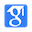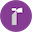## AI generates interpretation videos

AI extracts and analyses the key points of the paper to generate videos automaticallyGo Generating

## AI Traceability

AI parses the academic lineage of this thesisGenerate MRT

## AI Insight

AI extracts a summary of this paper

Weibo:
We describe how logical qubits are constructed in the surface code and show how the complete set of single logical qubit gates and the two-qubit logical CNOT are constructed, allowing us to implement quantum algorithms based on these logical qubits

# Surface codes: Towards practical large-scale quantum computation

PHYSICAL REVIEW A, no. 3 (2012)

Cited: 705|Views36
Full Text
Bibtex
Weibo

Abstract

This article provides an introduction to surface code quantum computing. We first estimate the size and speed of a surface code quantum computer. We then introduce the concept of the stabilizer, using two qubits, and extend this concept to stabilizers acting on a two-dimensional array of physical qubits, on which we implement the surface ...More

Code:

Data:

Introduction
• Quantum computation relies on the use of qubits, which are two-level quantum systems.
• The measure-Z and measure-X qubits that stabilize the surface code are operated in a very particular sequence, with one complete cycle shown in Figs.
Highlights
• Quantum computation relies on the use of qubits, which are two-level quantum systems
• We describe how logical qubits are constructed in the surface code and show how the complete set of single logical qubit gates and the two-qubit logical CNOT are constructed, allowing us to implement quantum algorithms based on these logical qubits
• We have only shown how to define a single logical qubit in our array; later we show how to make larger numbers of logical qubits within an array, which is done by creating more degrees of freedom within the array
• The simulations used to generate Fig. 4 include the following types of errors, occurring during the surface code cycle shown in Fig. 1: (1) attempting to perform a data qubit identity I, but instead performing a single-qubit operation X, Y, or Z, each occurring with probability p/3; (2) attempting to initialize a qubit to |g, but instead preparing |e with probability p; 11Actually, the surface code does not need to completely identify errors; it is sufficient that it identifies errors or chains of errors that are topologically equivalent to the actual errors, meaning any differences can be written as products of stabilizers
• A particular pattern of errors in time will suffer misidentifications following the same scaling as in Eq (12), so we find that we need roughly the same distance d in time as we do in space to maintain the minimum logical error rate, assuming the measurement error rate pM is comparable to the physical qubit error rate p
• XI we discussed the handling of errors when the surface code stabilizers are being manipulated, where we focused on errors occurring when an X-cut logical qubit is initialized in a Z L eigenstate
Results
• Following this error, when the surface code measurement cycle is completed, the state |ψ will be projected to an eigenstate of all the XaXbXcXd and ZaZbZcZd operator products.
• The state |ψZ clearly differs from |ψ , as the authors have phase flipped five data qubits, and as Z L cannot be written as a product of stabilizers, |ψZ is not trivially related to |ψ ; Z L manipulates a second degree of freedom in the array.
• The simulations used to generate Fig. 4 include the following types of errors, occurring during the surface code cycle shown in Fig. 1: (1) attempting to perform a data qubit identity I, but instead performing a single-qubit operation X , Y , or Z , each occurring with probability p/3; (2) attempting to initialize a qubit to |g , but instead preparing |e with probability p; 11Actually, the surface code does not need to completely identify errors; it is sufficient that it identifies errors or chains of errors that are topologically equivalent to the actual errors, meaning any differences can be written as products of stabilizers.
• Edmonds’ matching algorithm maps changes detected in the stabilizer outcomes to physical qubit errors; the rate at which the algorithm makes mistakes, meaning it misidentifies the source of a particular error report, is displayed as PL, the number of X L errors appearing anywhere in the array, per surface code cycle.13 This error rate is plotted as a function of the per-step error rate p.
• Returning to the general error rate discussion, the authors see that the surface code is able to handle a per-step physical qubit error rate up to the threshold pth = 0.57%, while still preserving the integrity of logical states in the array.
Conclusion
• The authors have physical qubits which are being projectively measured on every surface code cycle, with errors being continuously detected and accounted for in software.
• In Fig. 9 we show how this can be done, by turning off a single measure-Z qubit and creating a hole, which we call a “Z-cut hole.” Turning off the measure-Z qubit means that we no longer measure its stabilizer, which creates two additional degrees of freedom in the surface code array.
Tables
• Table1: Trade-off between number of computational logical qubits and number of sequential and total Toffoli gate operations for factoring an N -bit number into its primes using Shor’s algorithm. Each line in the table corresponds to a different quantum circuit implementing the algorithm. The physical size of a circuit scales with the ratio of the total number of Toffoli gates to the number of sequential Toffoli gates
• Table2: Translation between the “traditional” language used in the published literature (e.g., from Ref. ) and the terminology used here
• Table3: Eigenstates of the two-qubit operators ZaZb and XaXb. The four eigenstates are the Bell states for this system
• Table4: TL logic circuit outcomes and commutation relations for TL with Z L and X L. The measurement MZ indicates which state is output from the circuit
• Table5: Table of measurement outcomes for the Soperation and MZ and MX measurement. For compactness, the state |ψ has been dropped from inside the MX measurement brackets. The second row shows the angle θ for the input state |g + exp(iθ)|e after application of Z , X , or Yerrors to the state |Y
• Table6: Eigenstates for the four-qubit stabilizers ZaZbZcZd and XaXbXcXd
• Table7: Table of measurement outcomes for the Toperation and MZ and MX measurements. Columns show ideal input state |A and after application of Z , X , or Yerrors. Entries with ∨ (“or”) yield one entry or the other, so approximately half the time succeed and the other half fail
Funding
• M.M. acknowledges support from an Elings Postdoctoral Fellowship
• A.G.F. acknowledges support from the Australian Research Council Centre of Excellence for Quantum Computation and Communication Technology (project number CE110001027), with support from the US National Security Agency and the US Army Research Office under contract number W911NF-08-1-0527, and by the Intelligence Advanced Research Projects Activity (IARPA) via Department of Interior National Business Center contract number D11PC20166
Reference
• We can make a rough estimate of the time and circuit size needed to factor a number with N = 2000 bits (600 decimal digits) using a circuit size scaling as in the first line of Table I and making assumptions about the physical qubit error rates and gate times; more details on this estimate are provided in Appendix M.3 This Shor’s algorithm implementation is constructed from ideas in Refs. [30–32] and involves a resource-intensive modular exponentiation that requires approximately 40N 3 ≈ 3 × 1011 sequential Toffoli gates. The modular exponentiation thus determines the total execution time for the factoring algorithm. A highly optimized version of this circuit  can complete each Toffoli gate in approximately three physical qubit measurement cycles. If we assume a physical qubit measurement time of 100 ns, it will take about 26.7 h to complete the exponentiation.Author0